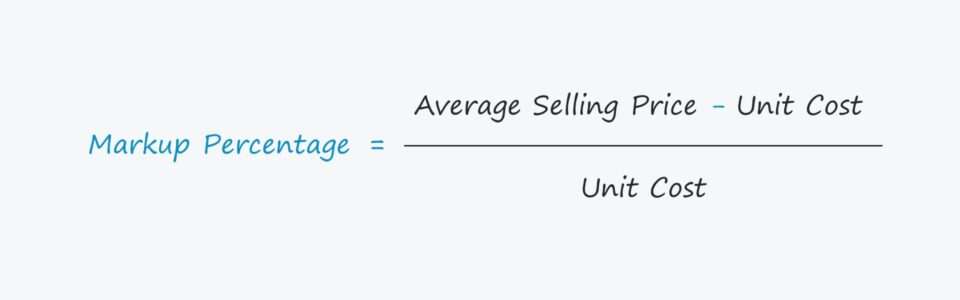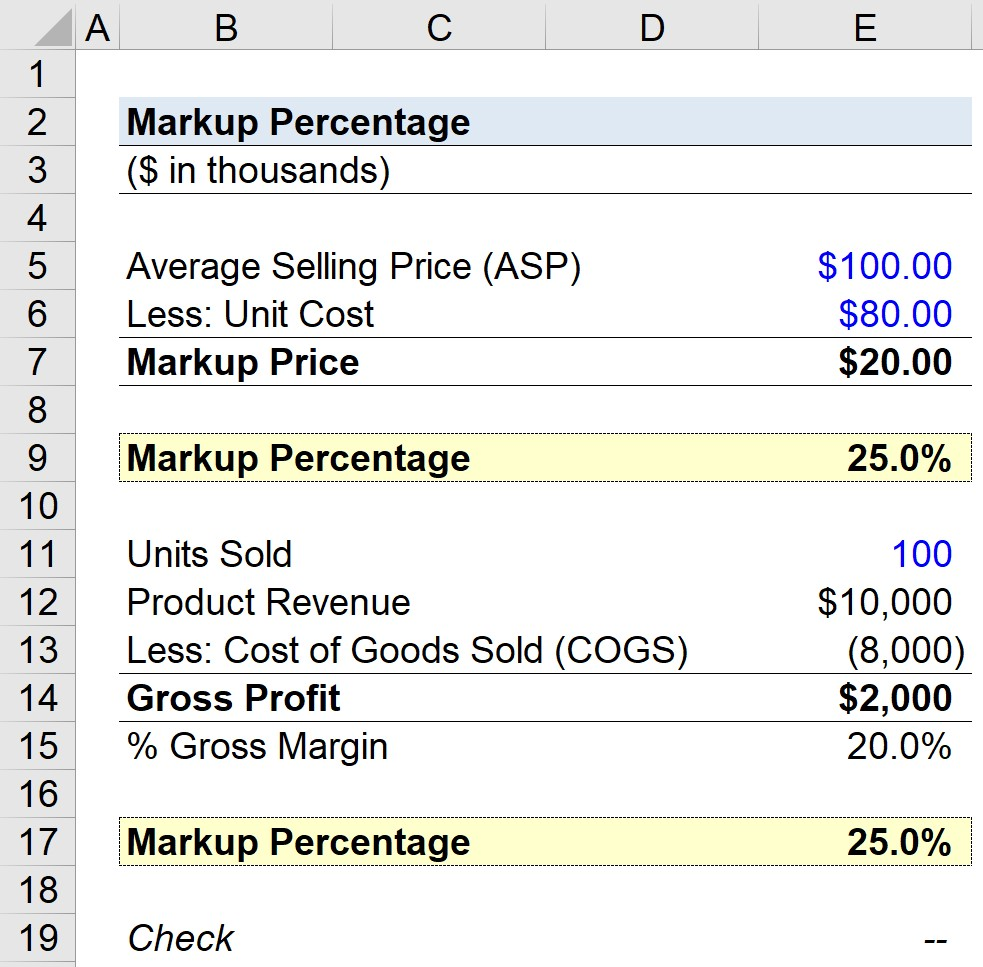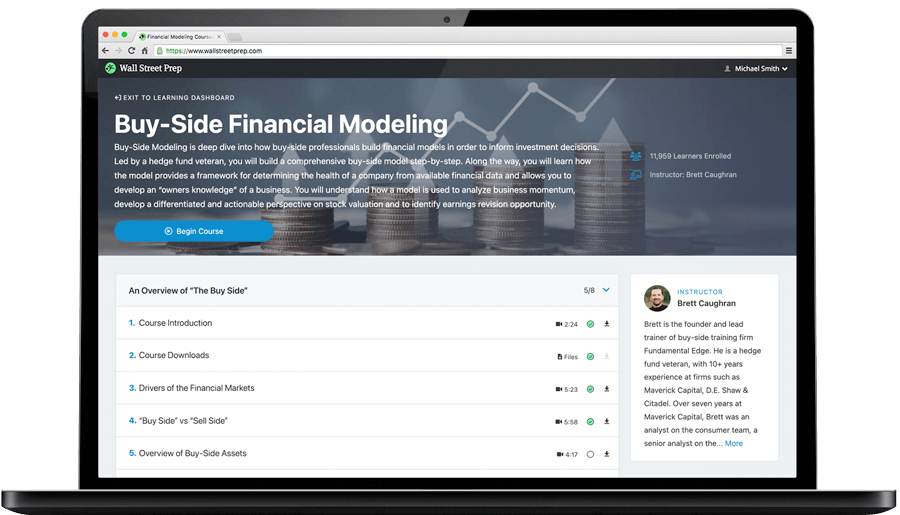Welcome to Wall Street Prep! Use code at checkout for 15% off.# Markup Percentage

Guide to Understanding the Markup Percentage## How to Calculate Markup Percentage?

The markup price is the difference between the average selling price (ASP) of a product and the corresponding unit cost, i.e. the cost of production on a per-unit basis.

###### Markup Price Formula

Markup Price = Average Selling Price Per Unit – Average Cost Per Unit

In practice, the markup price is typically calculated for internal uses and to help set prices.

All companies, irrespective of the industry they operate within, must eventually turn a profit in order to be able to sustain operations, meaning overhead costs and other operating expenses must all be covered sufficiently by revenue.

Further, one of the most influential decisions on a company’s profit margins is the pricing of its products/services.

But as a standalone metric, the markup price does not provide much insight, which is where the markup percentage comes in.

Given a markup price, calculating the markup percentage is a relatively straightforward process.

• Step 1: The markup price is calculated by subtracting the average cost per unit from the ASP
• Step 2: The average selling price (ASP) is simply subtracted by the unit cost and then divided by the unit cost
• Step 3: To convert the result into a percentage, the resulting figure must then be multiplied by 100

## Markup Percentage Formula

The formula for calculating the markup percentage is as follows.

###### Markup Percentage Formula
• Markup Percentage = (Average Selling Price – Unit Cost) ÷ Unit Cost

## Markup Percentage Calculator – Excel Template

We’ll now move to a modeling exercise, which you can access by filling out the form below.Submitting...

## Markup Percentage Calculation Example

Suppose a retail store sells its products for an average selling price (ASP) of \$100.00 each.

The unit costs associated with the production are \$80.00 per product.

• Average Selling Price (ASP) = \$100.00
• Unit Cost = \$80.00

Upon subtracting the unit cost from the average selling price (ASP), we arrive at a markup price of \$20.00 per unit.

• Markup Price = \$100.00 – \$80.00 = \$20.00

From the calculation above, we can see that the excess charged above the unit cost if \$20.00.

The next step is to convert our markup price to the markup percentage metric by dividing the markup price by the unit cost, which comes out as a markup of 25%.

• Markup Percentage = (\$100.00 – \$80.00) ÷ \$80.00 = 25%

## Markup vs. Margin: What is the Difference?

Hypothetically, let’s say that the retail store from the prior section sold 100,000 units in one month.

In this case, the company’s product revenue was \$10 million, while its cost of goods sold (COGS) was \$8 million.

• Product Revenue = \$10 million
• COGS = \$8 million

For illustrative purposes, we’ll ignore any non-production-related expense that could be embedded within COGS and focus solely on the products sold (and their markup).

The gross profit equals \$2 million, which we calculated by subtracting the COGS from the product revenue (and the gross margin is thus 20%).

• Gross Profit = \$10 million – \$8 million = \$2 million
• Gross Margin (%) = \$2 million ÷ \$10 million = 20%

Using an alternative approach, the markup percentage can be calculated by taking the gross profit and dividing it by the cost of goods sold (COGS).

The distinction between the gross margin and the markup percentage is that the gross margin is divided by revenue, whereas the markup percentage is divided by COGS.

The markup percentage of 25% confirms our calculation from earlier was correct.Step-by-Step Online Course

### Learn Buy-Side (Hedge Fund) Modeling

Led by a former hedge fund PM (Maverick, Citadel, DE Shaw, Schonfeld), this program begins where financial modeling training ends — with a deep-dive into how buy-side analysts build financial models to make key investment decisions.